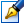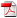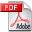##The approximate solving of integral equations by the Intelligent Software tools

 Author: Seiciuc Eleonora Degree: doctor of physics and mathematics Speciality: 01.01.09 - Mathematical cybernetics and operation research Year: 2008 Scientific advisers: Gheorghe Căpăţânădoctor, professor, Moldova State UniversityVladimir Zolotarevschidoctor habilitat, professor Institution: Scientific council:

### Status

The thesis was presented on the 17 September, 2008
Approved by NCAA on the 23 October, 2008

### Abstract0.40 Mb / in romanian

### Thesis

CZU 004:5.6.91; 519.62.25 Mb / in romanian
191 pages

### Keywords

Integral equations (EI), the spline-collocations method, the spline-quadratures method, Intelligent Information System (SII) for the approximate solving of integral equations Fredholm and Volterra of the second kind (SII_EI), the Base of Prototype of Kernels for compatibility (BPN_EI_COMP), the Base of Prototype of Kernels for collocations (BPN_EI_COL), the Integral Equations Solver (REI), decision support systems

### Summary

The transition to an informational society assumes an increase of computers’ productivity in information technologies’ application at the solving of a wide class of actual applied problems. Significant parts from them are the problems, which mathematical models are reduced to various classes of the integral equations.

In the dissertation are developed and theoretical substantiate the methods of spline-collocations and spline-quadratures; also, it is developed and implement the Intelligent Information System for the approximate solving of integral equation (SII_EI) Fredholm and Volterra of the second kind, based on following received theoretical and applicative results:

• development of methods of spline-collocations and spline-quadratures for the approximate solving integral equations Fredholm and Volterra of the second kind;
• a theoretical substantiation in the space of continuous functions of the developed methods;
• an establishment of principles and architecture of development SII_EI;
• development SII_EI based on the above described architecture for solving integral equations of the second kind.
Based on offered kernels for integral equations (more than 1300 types) were created: the Base of Prototype of Kernels of integral equations (BPN_EI_COMP) for checking the sufficient conditions of integral equations compatibility; the Base of Prototype of Kernels of integral equations (BPN_EI_COL) for method of spline-collocations application for solving the integral equations; the Integral Equations Solver (REI), accompanied by the convenient interface during the solving of integral equations.

It was developed a mathematical model for macroeconomics which determines the increased level of production funds, hence that necessary production level of consumer goods to be assured.

Both the solving solution of this problem based on developed intellectual system and the results of testing of impressive quantity of integrated equations, completely confirm the efficiency of the applied methodology to solve the integrated equations and developments of intelligent support system.

Also, it was proved the fact, that end users, assisted by the developed intelligent support system, can independently solve the integrated equations from corresponding application areas and develop own versions of intelligent systems for corresponding areas of work without obligatory consultations with experts from area of mathematics and computer science.